# Methods and formulas for potential capability measures in Normal Capability Analysis for Multiple Variables

## Cp

### Notation

TermDescription
USLUpper specification limit
LSLLower specification limit
TolerMultiplier of the sigma tolerance (Minitab uses 6 as the default value)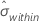Within-subgroup standard deviation

## Cp confidence interval bounds

The (1 -α) 100% confidence interval for Cp is calculated as follows: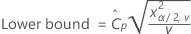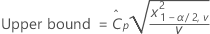where ν is calculated based on the method used to estimate σ2within:

• Pooled standard deviation: ν = Σ (ni- 1)
• Average moving range and Median moving range: ν ≈ k – Rspan + 1
• Square root of MSSD: ν = k - 1
• Rbar: ν = 0.9 k (n - 1)
• Sbar: ν = fn k (n - 1), where fn is the adjustment factor that varies with n, as shown in the following table.
n fn
2 0.88
3 0.92
4 0.94
5 0.95
6, 7 0.96
8, 9 0.97
10-17 0.98
18-64 0.99
65- 1.00

### Notation

TermDescription
χ2α,νThe α percentile of the chi-square distribution with ν degrees of freedom
αAlpha for the confidence level
νDegrees of freedom
σ2withinWithin-subgroup variance
ni The ith subgroup size
kNumber of samples
RspanLength of the moving range
nAverage sample size (Σ ni / k).

## CPL

### Notation

TermDescriptionProcess mean (estimated from the sample data or a historical value)
LSLLower specification limit
TolerMultiplier of the sigma tolerance (Minitab uses 6 as the default value)Within-subgroup standard deviation

## CPU

### Notation

TermDescription
USLUpper specification limitProcess mean (estimated from the sample data or a historical value)
TolerMultiplier of the sigma tolerance (Minitab uses 6 as the default value)Within-subgroup standard deviation

## Cpk confidence interval bounds

The (1 -α) 100% confidence interval for Cpk is calculated as follows:

### Notation

TermDescription
NThe total number of observations
αAlpha for the confidence level
vThe degrees of freedom based on the method used to estimate σ2within (for information on the calculation of v, see the section on Cp confidence interval bounds)
TolerMultiplier of the sigma tolerance (Minitab uses 6 as the default value)
Z1-α/2The 1-α/2 percentile from the standard normal distribution

## CCpk

Minitab calculates CCpk based on known values of LSL, USL, and T.

Known value CCpk
USL only
LSL only
LSL and USL

where: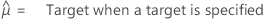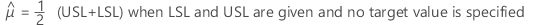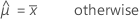### Notation

TermDescription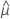Estimator for the population mean
USLUpper specification limit
LSLLower specification limit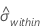Within-subgroup standard deviationAverage of the observations
TolerMultiplier of the sigma tolerance (Minitab uses 6 as the default value)
By using this site you agree to the use of cookies for analytics and personalized content.  Read our policy